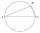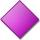RT 10

Area of right triangle is 84 cm2 and one of its cathethus is a=10 cm. Calculate perimeter of the triangle ABC.

Result

x =  46.35 cm

Solution:Try calculation via our triangle calculator.

Leave us a comment of example and its solution (i.e. if it is still somewhat unclear...):Be the first to comment!To solve this example are needed these knowledge from mathematics:

Pythagorean theorem is the base for the right triangle calculator.

Next similar examples:

1. Right triangle ABCCalculate the perimeter and area of a right triangle ABC, if you know the length of legs 4 cm 5.5 cm and 6.8 cm is hypotenuse.
2. HypotenuseCalculate the length of the hypotenuse of a right triangle if the length of one leg is 4 cm and its content area is 16 square centimeters.
3. TriangleCalculate the area of right triangle ΔABC, if one leg is long 14 and its opposite angle is 59°.
4. Circle - simpleCalculate the area of a circle in dm2, if its circumference is 31.4 cm.
5. Rhombus 4Circumference of the rhombus is 44 cm, its height is 89 mm long. Calculate its content area.
6. Trapezium 2Trapezium has an area of 24 square cms. How many different trapeziums can be formed ?
7. Four ropesTV transmitter is anchored at a height of 44 meters by four ropes. Each rope is attached at a distance of 55 meters from the heel of the TV transmitter. Calculate how many meters of rope were used in the construction of the transmitter. At each attachment.
8. Chord circleThe circle to the (S, r = 8 cm) are different points A, B connected segment /AB/ = 12 cm. AB mark the middle of S'. Calculate |SS'|. Make the sketch.
9. Broken treeThe tree is broken at 4 meters above the ground and the top of the tree touches the ground at a distance of 5 from the trunk. Calculate the original height of the tree.
10. WindbreakA tree at a height of 3 meters broke in the windbreak. Its peak fell 4.5 m from the tree. How tall was the tree?The ladder has a length 3.5 meters. He is leaning against the wall so that his bottom end is 2 meters away from the wall. Determine the height of the ladder.Decide whether trio of numbers is the side of a right triangle: 26,24,10.The tree was 35 meters high. The tree broke at a height of 10 m above the ground. Top but does not fall off it refuted on the ground. How far from the base of the tree lay its peak?Is right that in any right triangle height is less or equal half of the hypotenuse?Calculate the diameter and radius of the circle if it has length 52.45 cm.Wheel in traction tower has a diameter 5 m. How many meters will perform an elevator cabin if wheel rotates in the same direction 49 times?In the park there is a large circular flowerbed with a diameter of 12 m. Jakub circulated him ten times and the smaller Vojtoseven times. How many meters each went by and how many meters did Jakub run more than Vojta?